# Lazy java

Aug. 9, 2017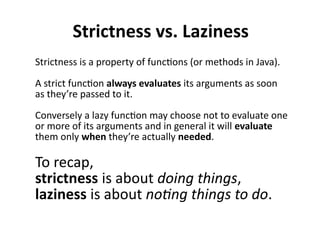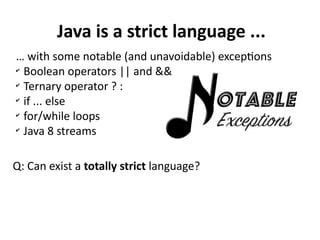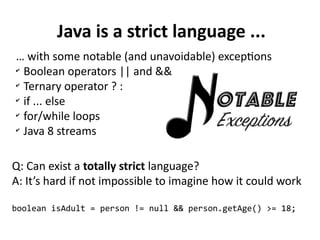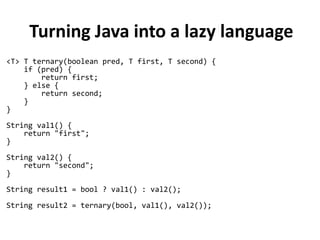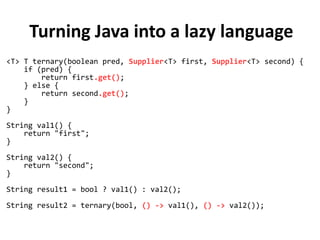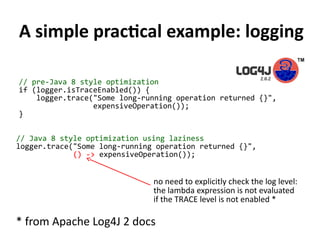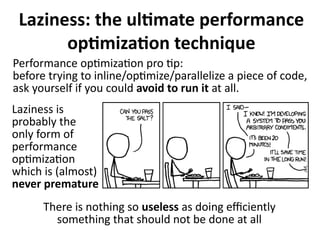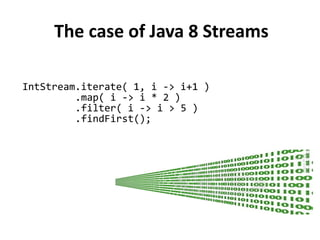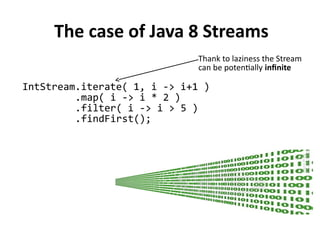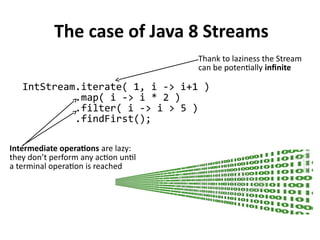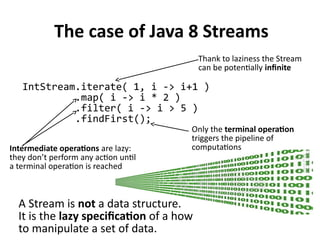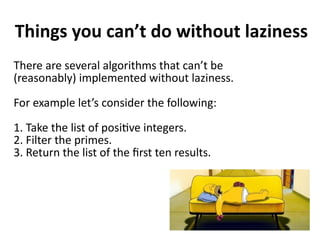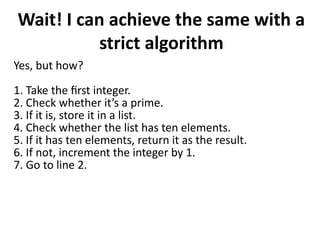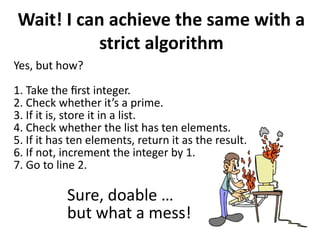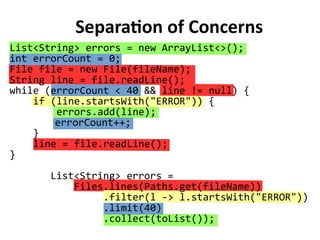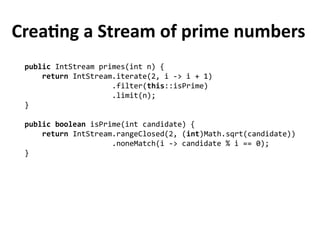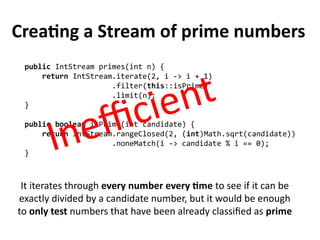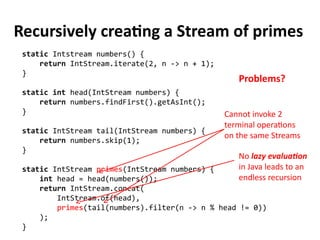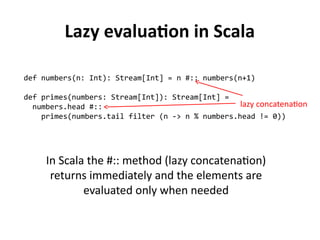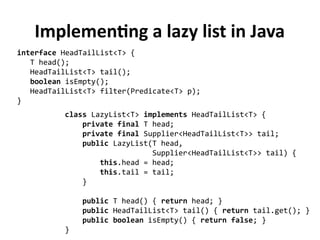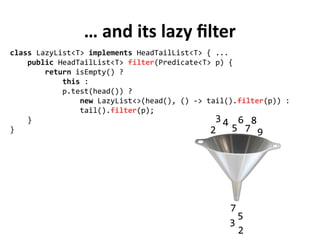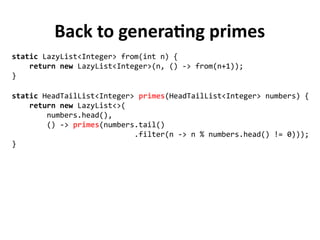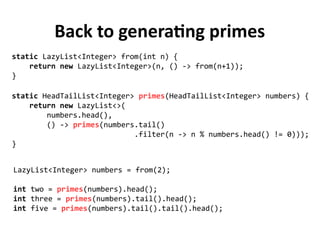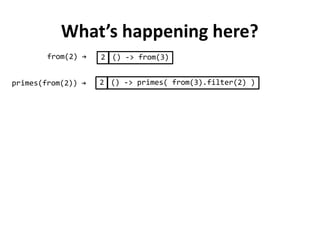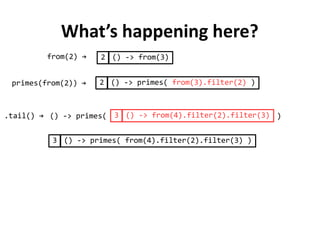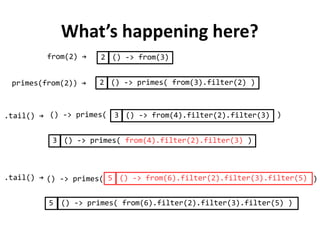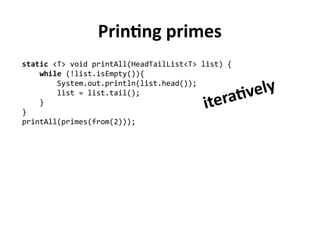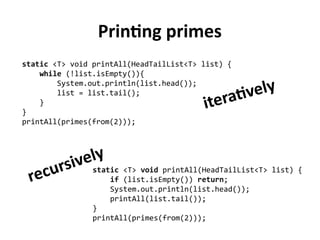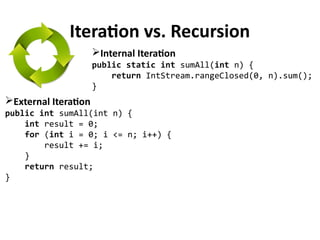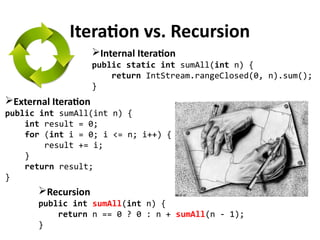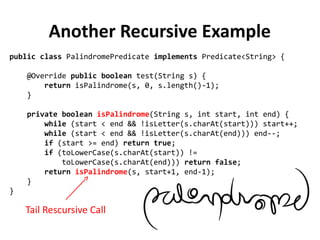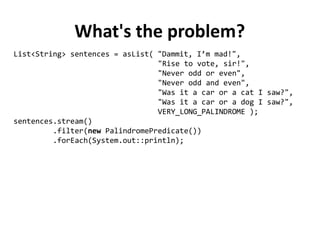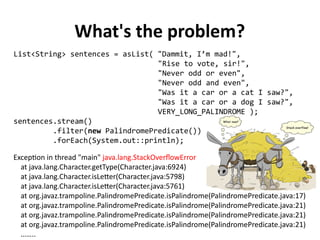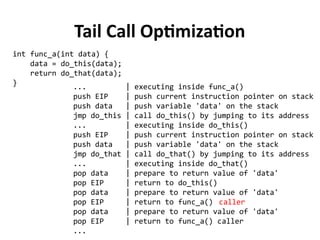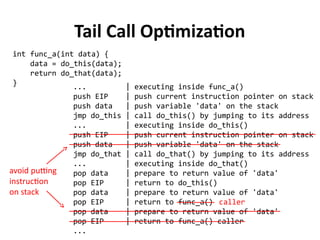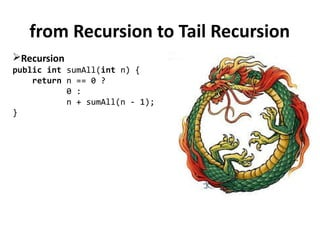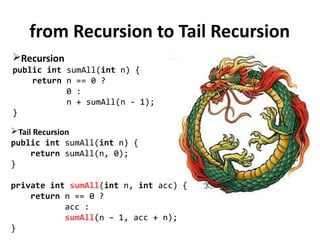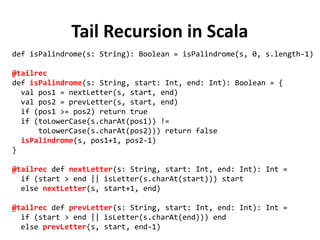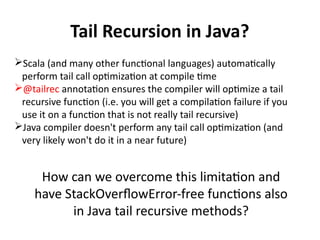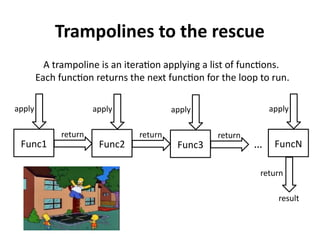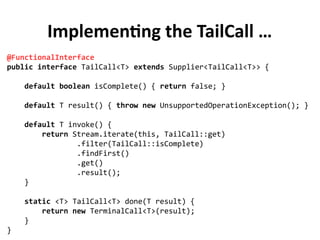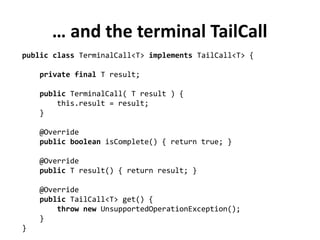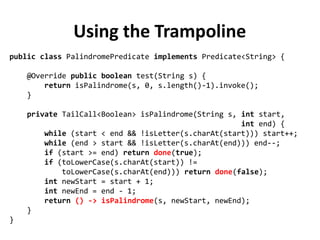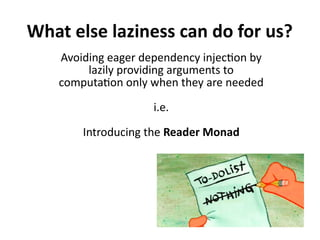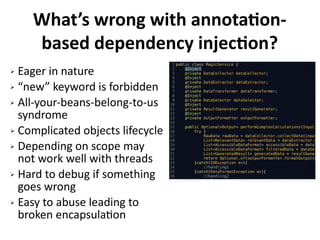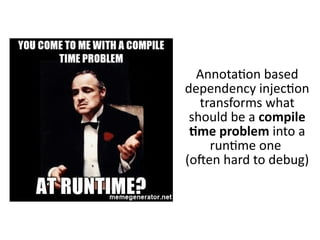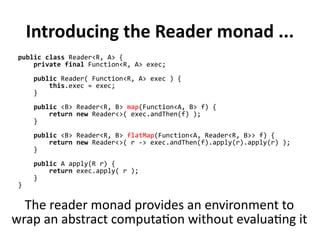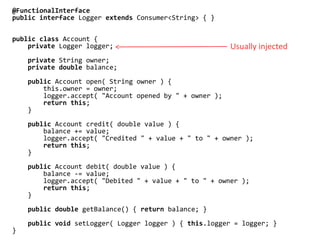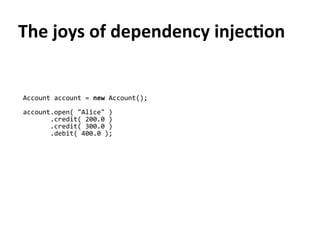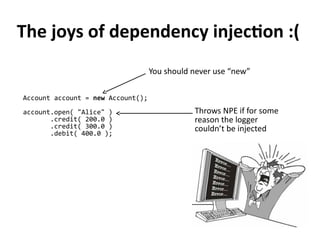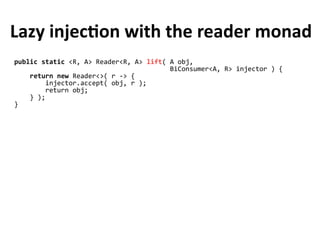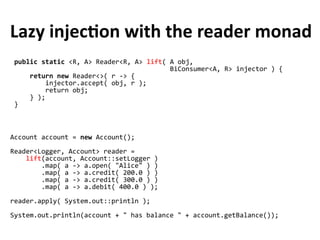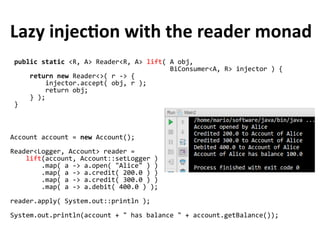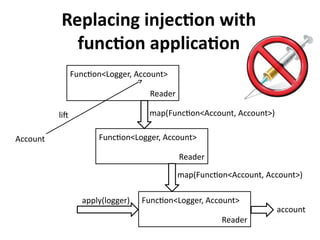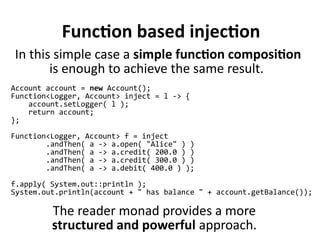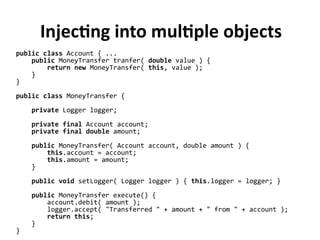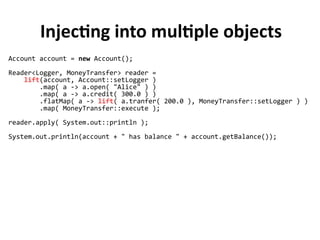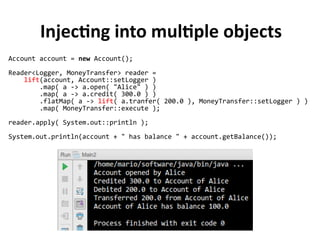1 of 66

### Lazy java

• 1. λazy by Mario Fusco Red Hat – Principal Software Engineer @mariofusco
• 2. Lazy Evaluation Lazy evaluation (or call-by-name) is an evaluation strategy which delays the evaluation of an expression until its value is needed I know what to do. Wake me up when you really need it
• 3. Strictness vs. Laziness Strictness is a property of functions (or methods in Java). A strict function always evaluates its arguments as soon as they’re passed to it. Conversely a lazy function may choose not to evaluate one or more of its arguments and in general it will evaluate them only when they’re actually needed. To recap, strictness is about doing things, laziness is about noting things to do.
• 4. Java is a strict language ... … with some notable (and unavoidable) exceptions ✔ Boolean operators || and && ✔ Ternary operator ? : ✔ if ... else ✔ for/while loops ✔ Java 8 streams Q: Can exist a totally strict language?
• 5. Java is a strict language ... … with some notable (and unavoidable) exceptions ✔ Boolean operators || and && ✔ Ternary operator ? : ✔ if ... else ✔ for/while loops ✔ Java 8 streams Q: Can exist a totally strict language? A: It’s hard if not impossible to imagine how it could work boolean isAdult = person != null && person.getAge() >= 18;
• 6. Turning Java into a lazy language <T> T ternary(boolean pred, T first, T second) { if (pred) { return first; } else { return second; } } String val1() { return "first"; } String val2() { return "second"; } String result1 = bool ? val1() : val2(); String result2 = ternary(bool, val1(), val2());
• 7. Turning Java into a lazy language <T> T ternary(boolean pred, Supplier<T> first, Supplier<T> second) { if (pred) { return first.get(); } else { return second.get(); } } String val1() { return "first"; } String val2() { return "second"; } String result1 = bool ? val1() : val2(); String result2 = ternary(bool, () -> val1(), () -> val2());
• 8. A simple practical example: logging // pre-Java 8 style optimization if (logger.isTraceEnabled()) { logger.trace("Some long-running operation returned {}", expensiveOperation()); }
• 9. A simple practical example: logging // pre-Java 8 style optimization if (logger.isTraceEnabled()) { logger.trace("Some long-running operation returned {}", expensiveOperation()); } // Java 8 style optimization using laziness logger.trace("Some long-running operation returned {}", () -> expensiveOperation()); * from Apache Log4J 2 docs no need to explicitly check the log level: the lambda expression is not evaluated if the TRACE level is not enabled *
• 10. Laziness: the ultimate performance optimization technique Performance optimization pro tip: before trying to inline/optimize/parallelize a piece of code, ask yourself if you could avoid to run it at all. Laziness is probably the only form of performance optimization which is (almost) never premature There is nothing so useless as doing efficiently something that should not be done at all
• 11. The case of Java 8 Streams IntStream.iterate( 1, i -> i+1 ) .map( i -> i * 2 ) .filter( i -> i > 5 ) .findFirst();
• 12. The case of Java 8 Streams IntStream.iterate( 1, i -> i+1 ) .map( i -> i * 2 ) .filter( i -> i > 5 ) .findFirst(); Thank to laziness the Stream can be potentially infinite
• 13. The case of Java 8 Streams IntStream.iterate( 1, i -> i+1 ) .map( i -> i * 2 ) .filter( i -> i > 5 ) .findFirst(); Thank to laziness the Stream can be potentially infinite Intermediate operations are lazy: they don’t perform any action until a terminal operation is reached
• 14. The case of Java 8 Streams IntStream.iterate( 1, i -> i+1 ) .map( i -> i * 2 ) .filter( i -> i > 5 ) .findFirst(); Thank to laziness the Stream can be potentially infinite Intermediate operations are lazy: they don’t perform any action until a terminal operation is reached Only the terminal operation triggers the pipeline of computations A Stream is not a data structure. It is the lazy specification of a how to manipulate a set of data.
• 15. Things you can’t do without laziness There are several algorithms that can’t be (reasonably) implemented without laziness. For example let’s consider the following: 1. Take the list of positive integers. 2. Filter the primes. 3. Return the list of the first ten results.
• 16. Wait! I can achieve the same with a strict algorithm Yes, but how? 1. Take the first integer. 2. Check whether it’s a prime. 3. If it is, store it in a list. 4. Check whether the list has ten elements. 5. If it has ten elements, return it as the result. 6. If not, increment the integer by 1. 7. Go to line 2.
• 17. Wait! I can achieve the same with a strict algorithm Yes, but how? 1. Take the first integer. 2. Check whether it’s a prime. 3. If it is, store it in a list. 4. Check whether the list has ten elements. 5. If it has ten elements, return it as the result. 6. If not, increment the integer by 1. 7. Go to line 2. Sure, doable … but what a mess!
• 18. Laziness lets us separate the description of an expression from the evaluation of that expression Laziness is an enabler for separation of concerns
• 19. List<String> errors = Files.lines(Paths.get(fileName)) .filter(l -> l.startsWith("ERROR")) .limit(40) .collect(toList()); Separation of Concerns List<String> errors = new ArrayList<>(); int errorCount = 0; File file = new File(fileName); String line = file.readLine(); while (errorCount < 40 && line != null) { if (line.startsWith("ERROR")) { errors.add(line); errorCount++; } line = file.readLine(); }
• 20. Cool! Now I know: I will use a Stream also for prime numbers
• 21. Cool! Now I know: I will use a Stream also for prime numbers Let’s give this a try ...
• 22. Creating a Stream of prime numbers public IntStream primes(int n) { return IntStream.iterate(2, i -> i + 1) .filter(this::isPrime) .limit(n); } public boolean isPrime(int candidate) { return IntStream.rangeClosed(2, (int)Math.sqrt(candidate)) .noneMatch(i -> candidate % i == 0); }
• 23. Creating a Stream of prime numbers public IntStream primes(int n) { return IntStream.iterate(2, i -> i + 1) .filter(this::isPrime) .limit(n); } public boolean isPrime(int candidate) { return IntStream.rangeClosed(2, (int)Math.sqrt(candidate)) .noneMatch(i -> candidate % i == 0); } It iterates through every number every time to see if it can be exactly divided by a candidate number, but it would be enough to only test numbers that have been already classified as prime Inefficient
• 24. Recursively creating a Stream of primes static Intstream numbers() { return IntStream.iterate(2, n -> n + 1); } static int head(IntStream numbers) { return numbers.findFirst().getAsInt(); } static IntStream tail(IntStream numbers) { return numbers.skip(1); } static IntStream primes(IntStream numbers) { int head = head(numbers()); return IntStream.concat( IntStream.of(head), primes(tail(numbers).filter(n -> n % head != 0)) ); } Cannot invoke 2 terminal operations on the same Streams Problems? No lazy evaluation in Java leads to an endless recursion
• 25. Lazy evaluation in Scala def numbers(n: Int): Stream[Int] = n #:: numbers(n+1) def primes(numbers: Stream[Int]): Stream[Int] = numbers.head #:: primes(numbers.tail filter (n -> n % numbers.head != 0)) lazy concatenation In Scala the #:: method (lazy concatenation) returns immediately and the elements are evaluated only when needed
• 27. … and its lazy filter class LazyList<T> implements HeadTailList<T> { ... public HeadTailList<T> filter(Predicate<T> p) { return isEmpty() ? this : p.test(head()) ? new LazyList<>(head(), () -> tail().filter(p)) : tail().filter(p); } } 2 3 4 5 6 7 8 9 2 3 5 7
• 28. Back to generating primes static HeadTailList<Integer> primes(HeadTailList<Integer> numbers) { return new LazyList<>( numbers.head(), () -> primes(numbers.tail() .filter(n -> n % numbers.head() != 0))); } static LazyList<Integer> from(int n) { return new LazyList<Integer>(n, () -> from(n+1)); }
• 29. Back to generating primes static HeadTailList<Integer> primes(HeadTailList<Integer> numbers) { return new LazyList<>( numbers.head(), () -> primes(numbers.tail() .filter(n -> n % numbers.head() != 0))); } static LazyList<Integer> from(int n) { return new LazyList<Integer>(n, () -> from(n+1)); } LazyList<Integer> numbers = from(2); int two = primes(numbers).head(); int three = primes(numbers).tail().head(); int five = primes(numbers).tail().tail().head();
• 30. LazyList of primes under the hood from(2) → 2 () -> from(3) 2 () -> primes( from(3).filter(2) )primes(from(2)) →
• 31. LazyList of primes under the hood from(2) → 2 () -> from(3) 2 () -> primes( from(3).filter(2) ) 3 () -> from(4).filter(2).filter(3)() -> primes( ) 3 () -> primes( from(4).filter(2).filter(3) ) primes(from(2)) → .tail() →
• 32. LazyList of primes under the hood from(2) → 2 () -> from(3) 2 () -> primes( from(3).filter(2) ) 3 () -> from(4).filter(2).filter(3)() -> primes( ) 3 () -> primes( from(4).filter(2).filter(3) ) 5 () -> from(6).filter(2).filter(3).filter(5)() -> primes( ) 5 () -> primes( from(6).filter(2).filter(3).filter(5) ) primes(from(2)) → .tail() → .tail() →
• 33. Printing primes static <T> void printAll(HeadTailList<T> list) { while (!list.isEmpty()){ System.out.println(list.head()); list = list.tail(); } } printAll(primes(from(2))); iteratively
• 34. Printing primes static <T> void printAll(HeadTailList<T> list) { while (!list.isEmpty()){ System.out.println(list.head()); list = list.tail(); } } printAll(primes(from(2))); static <T> void printAll(HeadTailList<T> list) { if (list.isEmpty()) return; System.out.println(list.head()); printAll(list.tail()); } printAll(primes(from(2))); iteratively recursively
• 35. Iteration vs. Recursion External Iteration public int sumAll(int n) { int result = 0; for (int i = 0; i <= n; i++) { result += i; } return result; } Internal Iteration public static int sumAll(int n) { return IntStream.rangeClosed(0, n).sum(); }
• 36. Iteration vs. Recursion External Iteration public int sumAll(int n) { int result = 0; for (int i = 0; i <= n; i++) { result += i; } return result; } Recursion public int sumAll(int n) { return n == 0 ? 0 : n + sumAll(n - 1); } Internal Iteration public static int sumAll(int n) { return IntStream.rangeClosed(0, n).sum(); }
• 37. public class PalindromePredicate implements Predicate<String> { @Override public boolean test(String s) { return isPalindrome(s, 0, s.length()-1); } private boolean isPalindrome(String s, int start, int end) { while (start < end && !isLetter(s.charAt(start))) start++; while (start < end && !isLetter(s.charAt(end))) end--; if (start >= end) return true; if (toLowerCase(s.charAt(start)) != toLowerCase(s.charAt(end))) return false; return isPalindrome(s, start+1, end-1); } } Another Recursive Example Tail Rescursive Call
• 38. What's the problem? List<String> sentences = asList( "Dammit, I’m mad!", "Rise to vote, sir!", "Never odd or even", "Never odd and even", "Was it a car or a cat I saw?", "Was it a car or a dog I saw?", VERY_LONG_PALINDROME ); sentences.stream() .filter(new PalindromePredicate()) .forEach(System.out::println);
• 39. What's the problem? List<String> sentences = asList( "Dammit, I’m mad!", "Rise to vote, sir!", "Never odd or even", "Never odd and even", "Was it a car or a cat I saw?", "Was it a car or a dog I saw?", VERY_LONG_PALINDROME ); sentences.stream() .filter(new PalindromePredicate()) .forEach(System.out::println); Exception in thread "main" java.lang.StackOverflowError at java.lang.Character.getType(Character.java:6924) at java.lang.Character.isLetter(Character.java:5798) at java.lang.Character.isLetter(Character.java:5761) at org.javaz.trampoline.PalindromePredicate.isPalindrome(PalindromePredicate.java:17) at org.javaz.trampoline.PalindromePredicate.isPalindrome(PalindromePredicate.java:21) at org.javaz.trampoline.PalindromePredicate.isPalindrome(PalindromePredicate.java:21) at org.javaz.trampoline.PalindromePredicate.isPalindrome(PalindromePredicate.java:21) ……..
• 40. Tail Call Optimization int func_a(int data) { data = do_this(data); return do_that(data); } ... | executing inside func_a() push EIP | push current instruction pointer on stack push data | push variable 'data' on the stack jmp do_this | call do_this() by jumping to its address ... | executing inside do_this() push EIP | push current instruction pointer on stack push data | push variable 'data' on the stack jmp do_that | call do_that() by jumping to its address ... | executing inside do_that() pop data | prepare to return value of 'data' pop EIP | return to do_this() pop data | prepare to return value of 'data' pop EIP | return to func_a() pop data | prepare to return value of 'data' pop EIP | return to func_a() caller ... caller
• 41. Tail Call Optimization int func_a(int data) { data = do_this(data); return do_that(data); } ... | executing inside func_a() push EIP | push current instruction pointer on stack push data | push variable 'data' on the stack jmp do_this | call do_this() by jumping to its address ... | executing inside do_this() push EIP | push current instruction pointer on stack push data | push variable 'data' on the stack jmp do_that | call do_that() by jumping to its address ... | executing inside do_that() pop data | prepare to return value of 'data' pop EIP | return to do_this() pop data | prepare to return value of 'data' pop EIP | return to func_a() pop data | prepare to return value of 'data' pop EIP | return to func_a() caller ... caller avoid putting instruction on stack
• 42. from Recursion to Tail Recursion Recursion public int sumAll(int n) { return n == 0 ? 0 : n + sumAll(n - 1); }
• 43. from Recursion to Tail Recursion Recursion public int sumAll(int n) { return n == 0 ? 0 : n + sumAll(n - 1); } Tail Recursion public int sumAll(int n) { return sumAll(n, 0); } private int sumAll(int n, int acc) { return n == 0 ? acc : sumAll(n – 1, acc + n); }
• 44. Tail Recursion in Scala def isPalindrome(s: String): Boolean = isPalindrome(s, 0, s.length-1) @tailrec def isPalindrome(s: String, start: Int, end: Int): Boolean = { val pos1 = nextLetter(s, start, end) val pos2 = prevLetter(s, start, end) if (pos1 >= pos2) return true if (toLowerCase(s.charAt(pos1)) != toLowerCase(s.charAt(pos2))) return false isPalindrome(s, pos1+1, pos2-1) } @tailrec def nextLetter(s: String, start: Int, end: Int): Int = if (start > end || isLetter(s.charAt(start))) start else nextLetter(s, start+1, end) @tailrec def prevLetter(s: String, start: Int, end: Int): Int = if (start > end || isLetter(s.charAt(end))) end else prevLetter(s, start, end-1)
• 45. Tail Recursion in Java? Scala (and many other functional languages) automatically perform tail call optimization at compile time @tailrec annotation ensures the compiler will optimize a tail recursive function (i.e. you will get a compilation failure if you use it on a function that is not really tail recursive) Java compiler doesn't perform any tail call optimization (and very likely won't do it in a near future) How can we overcome this limitation and have StackOverflowError-free functions also in Java tail recursive methods?
• 46. Trampolines to the rescue A trampoline is an iteration applying a list of functions. Each function returns the next function for the loop to run. Func1 return apply Func2 return apply Func3 return apply FuncN apply … result return
• 47. Implementing the TailCall … @FunctionalInterface public interface TailCall<T> extends Supplier<TailCall<T>> { default boolean isComplete() { return false; } default T result() { throw new UnsupportedOperationException(); } default T invoke() { return Stream.iterate(this, TailCall::get) .filter(TailCall::isComplete) .findFirst() .get() .result(); } static <T> TailCall<T> done(T result) { return new TerminalCall<T>(result); } }
• 48. … and the terminal TailCall public class TerminalCall<T> implements TailCall<T> { private final T result; public TerminalCall( T result ) { this.result = result; } @Override public boolean isComplete() { return true; } @Override public T result() { return result; } @Override public TailCall<T> get() { throw new UnsupportedOperationException(); } }
• 49. Using the Trampoline public class PalindromePredicate implements Predicate<String> { @Override public boolean test(String s) { return isPalindrome(s, 0, s.length()-1).invoke(); } private TailCall<Boolean> isPalindrome(String s, int start, int end) { while (start < end && !isLetter(s.charAt(start))) start++; while (end > start && !isLetter(s.charAt(end))) end--; if (start >= end) return done(true); if (toLowerCase(s.charAt(start)) != toLowerCase(s.charAt(end))) return done(false); int newStart = start + 1; int newEnd = end - 1; return () -> isPalindrome(s, newStart, newEnd); } }
• 50. What else laziness can do for us? Avoiding eager dependency injection by lazily providing arguments to computation only when they are needed i.e. Introducing the Reader Monad
• 51. What’s wrong with annotation- based dependency injection? ➢ Eager in nature ➢ “new” keyword is forbidden ➢ All-your-beans-belong-to-us syndrome ➢ Complicated objects lifecycle ➢ Depending on scope may not work well with threads ➢ Hard to debug if something goes wrong ➢ Easy to abuse leading to broken encapsulation
• 52. Annotation based dependency injection transforms what should be a compile time problem into a runtime one (often hard to debug)